Friday, October 22, 2021
Home > Latest Announcement > NMTC 2018 Question Papers With Solutions Sub Junior Level

# NMTC 2018 Question Papers With Solutions Sub Junior Level# NMTC 2018 Question Papers with Solutions Sub Junior Level

NMTC is an acronym for National Mathematics Talent Contest. NMTC is conducted by AMTI (Association of Mathematics Teachers of India) to identify the talent of the students in Mathematics. In this post, NMTC 2018 Question Papers with Solutions Sub Junior Level is published.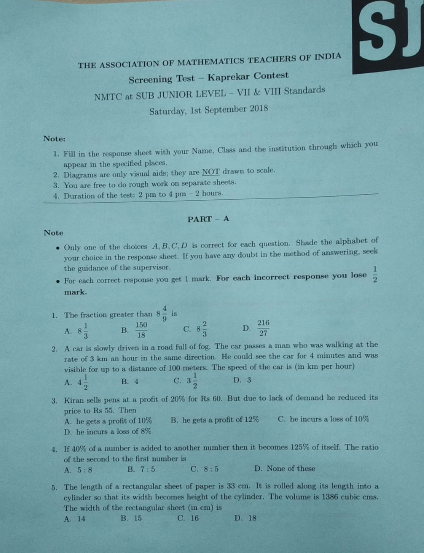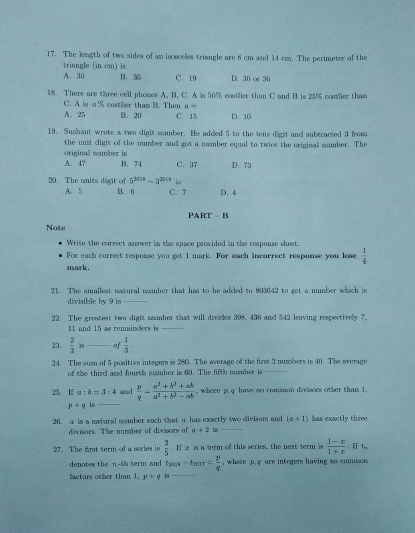Part A: Instruction:

Only One of the choices A, B, C, D is correct. For each correct response, you get 1 mark. For each incorrect response, you lose 1/2 mark. NMTC 2018 Question Papers with Solutions Sub Junior Level

NMTC 2018 Paper For Sub Junior Level Ques No 1:

The fraction greater thanis

Options:

A.B. 150/18

C.D. 216/27

Solution: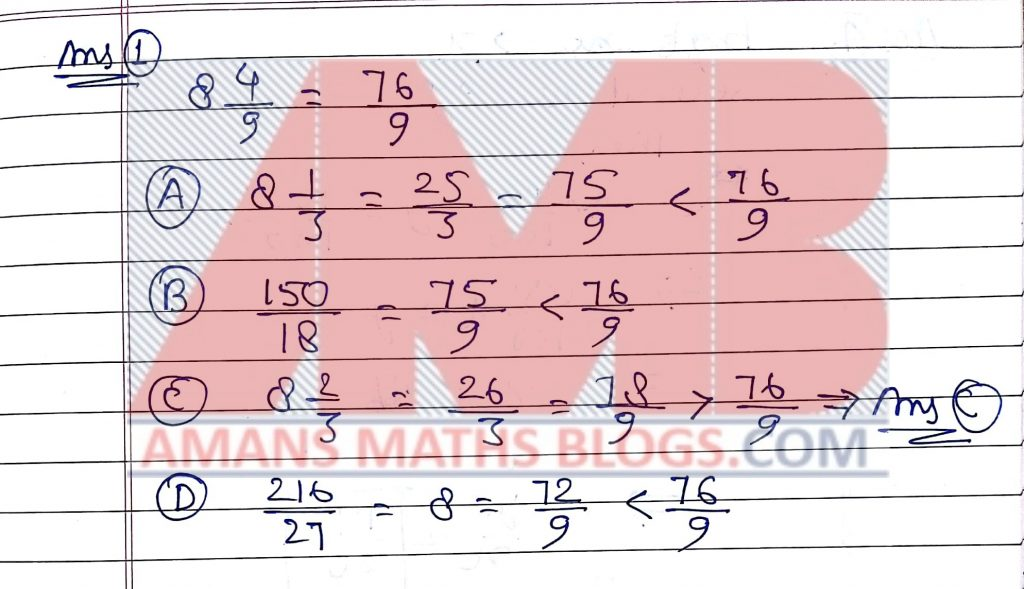NMTC 2018 Paper For Sub Junior Level Ques No 2:

A car is slowly driven in the road full of fog. The car passes a man who was walking at the rate of 3 km an hour in the same direction. He could see the car for 4 minutes and was visible for up to a distance of 100 meters. The speed of the car is (in km per hour)

Options:

A.B. 4

C.D. 3

Solution: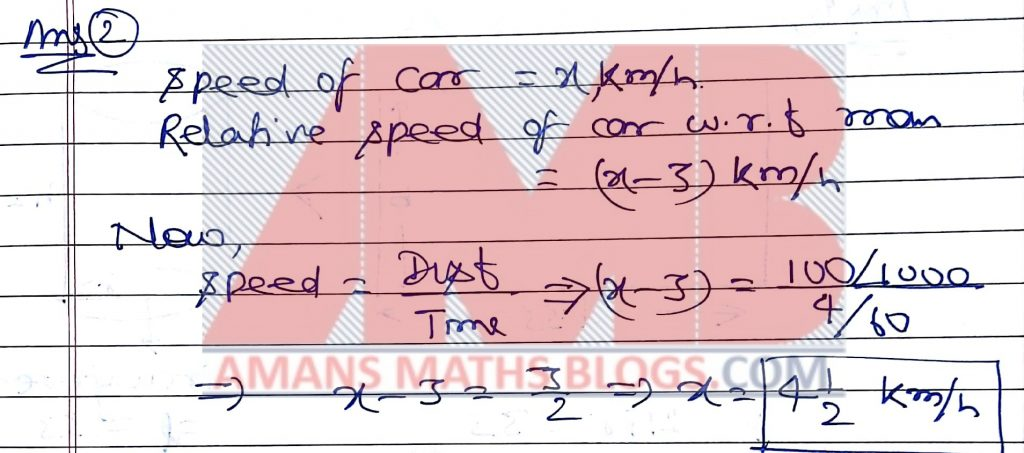NMTC 2018 Paper For Sub Junior Level Ques No 3:

Kiran sells pens at a profit of 20 % for Rs 60. But due to lack of demand he reduced its price to Rs. 55. Then

Options:

A. He gets a profit of 10%

B. He gets a profit of 12%

C. He incurs a loss of 10%

D. He incurs a loss of 10%

Solution:NMTC 2018 Paper For Sub Junior Level Ques No 4:

If 40% of a number is added to another number then it becomes 125% of itself. The ratio of the second to the first number is

Options:

A. 5 : 8

B. 7 : 5

C. 8 : 5

D. None of these

Solution: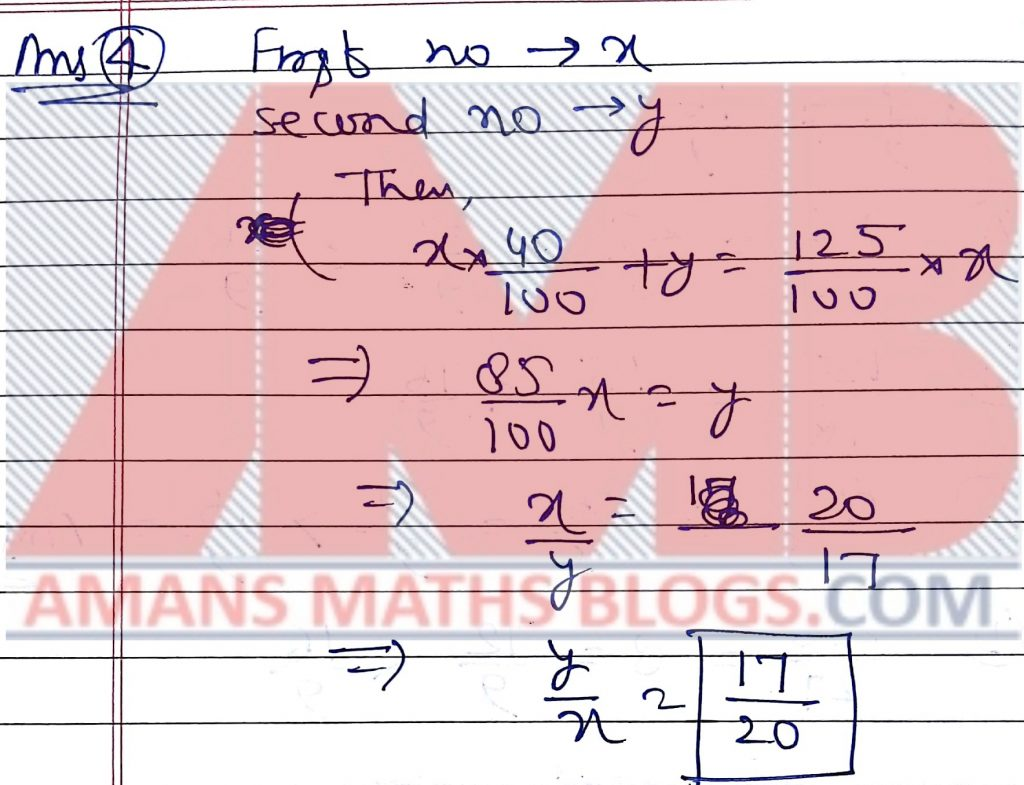NMTC 2018 Paper For Sub Junior Level Ques No 5:

The length of a rectangular sheet of paper is 33 cm. It is rolled along along its length into a cylinder so that its width becomes height of the cylinder. The volume of the cylinder is 1386 cubic cms. The width of the rectangular sheet ( in cm ) is

Options:

A. 14

B. 15

C. 16

D. 18

Solution:NMTC 2018 Paper For Sub Junior Level Ques No 6:

If 1/(1 * 2) + 1/(2 * 3) + 1/(3 * 4) + … + 1/{n * (n + 1)} = 19/20

Options:

A. 18

B. 19

C. 20

D. 25

Solution:NMTC 2018 Paper For Sub Junior Level Ques No 7:

a and b are natural numbers. If 9a2 = 12a + 96 and b2 = 2b + 3, the value of 2018(a + b) is

Options:

A. 14226

B. 14128

C. 14126

D. 14246

Solution: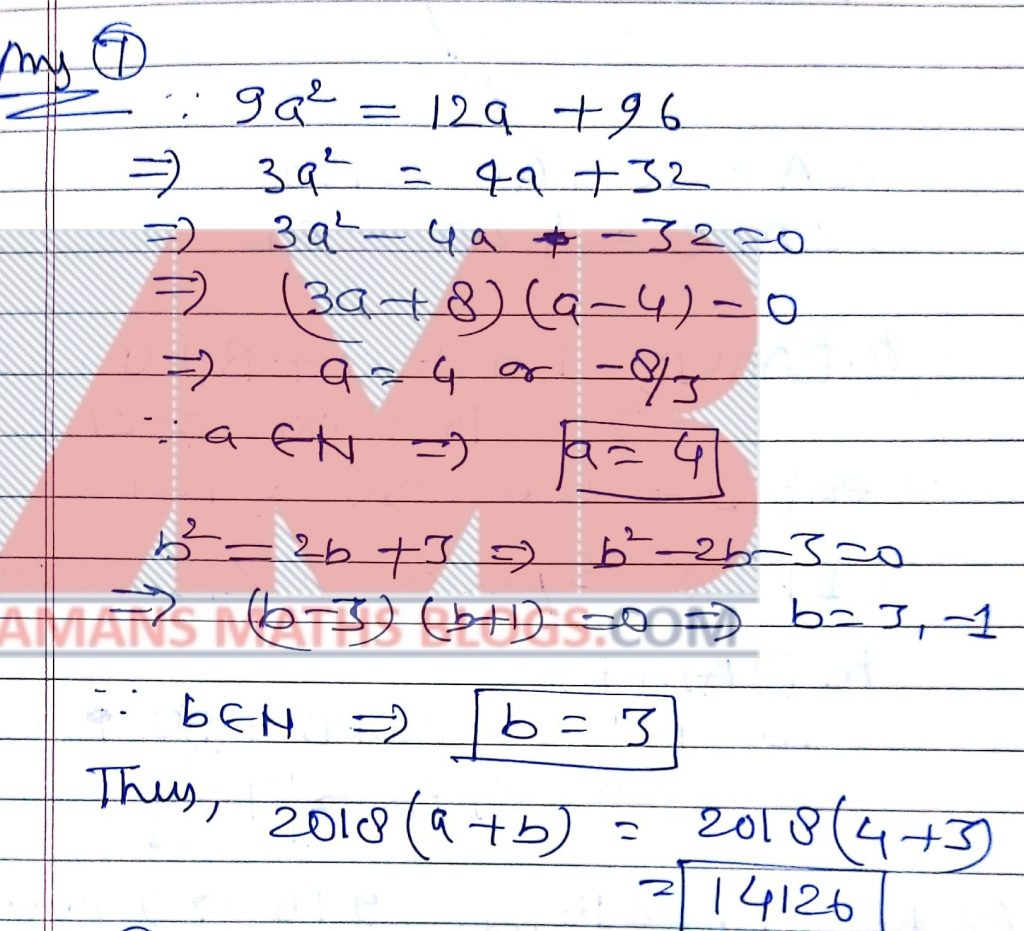NMTC 2018 Paper For Sub Junior Level Ques No 8:

Shanti has three daughters. The average age of them is 15 years. Their ages are in the ratio 3 : 5 : 7. The age of  youngest age is (in years)

Options:

A. 8

B. 9

C. 10

D. 12

Solution: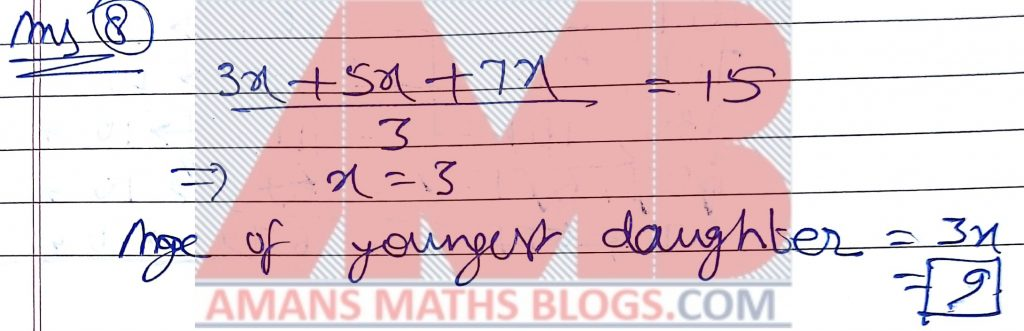NMTC 2018 Paper For Sub Junior Level Ques No 9:

In the adjoining figure, ABCD is a quadrilateral. The bisector of the angle B and the exterior angle at D meet at P. Given angle C = 80 degree, angle ADC = (1/2)A  and A = C + 40 degree, then the angle DPB is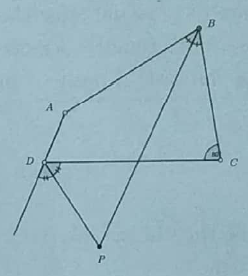Options:

A. 50

B. 60

C. 70

D. 80

Solution: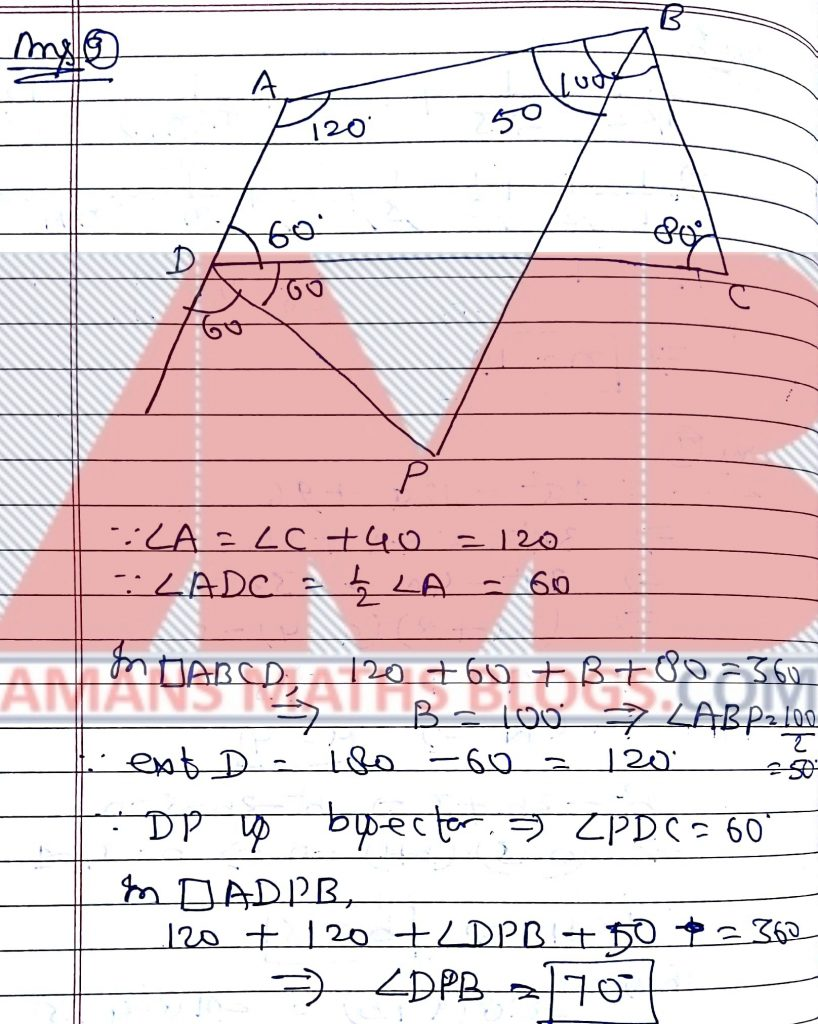NMTC 2018 Paper For Sub Junior Level Ques No 10:

The number of 3-digit numbers which contains 6 and 7 is

ptions:

A. 52

B. 60

C. 62

D. 64

Solution:NMTC 2018 Paper For Sub Junior Level Ques No 11:

The difference between the biggest and the smallest three digit numbers each of which has different digits is

Options:

A. 864

B. 875

C. 885

D. 895

Solution: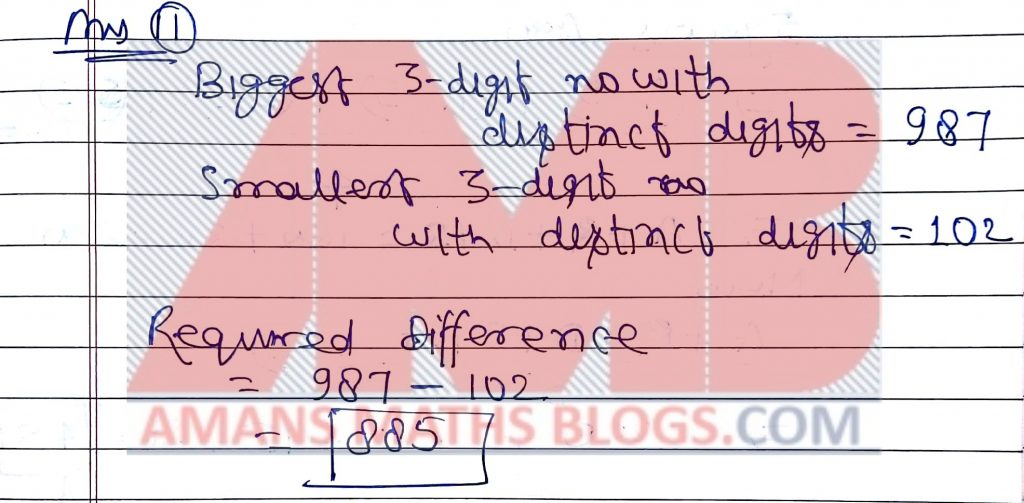NMTC 2018 Paper For Sub Junior Level Ques No 12:

If 3x + 1 = 2y – 1 = 5z + 3 = 7w + 1 = 15, then the value of 6x – 3y + 5z – 8w is

Options:

A. 1

B. 2

C. 3

D. None of these

Solution:NMTC 2018 Paper For Sub Junior Level Ques No 13:

Five years ago the average of Aruna, Roy, David and Salman is 45 years. Sita joins them now. The average age of all the five now is 49 years. The present age of Sita is (in years)

Options:

A. 45

B. 43

C. 51

D. 48

Solution: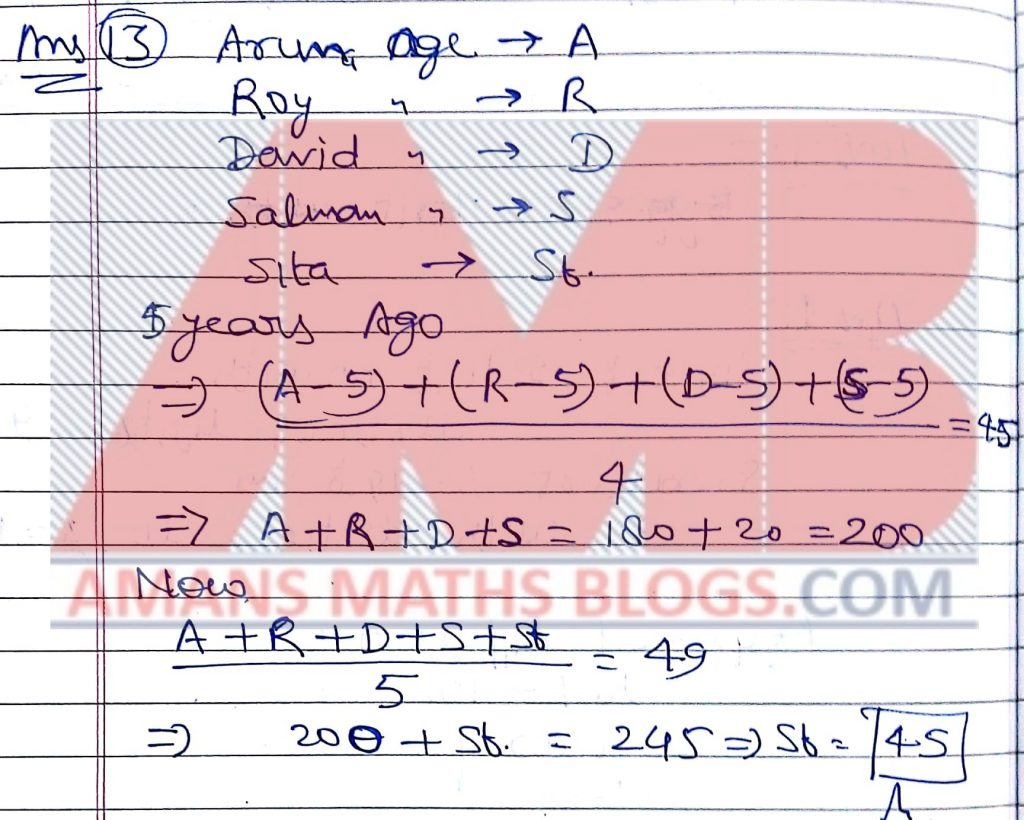NMTC 2018 Paper For Sub Junior Level Ques No 14:

The fraction B/(3x – 1) is subtracted from the fraction A/(2x + 3). The resulting fraction is -11/(2x + 3)(3x – 1). Then, A + B is

Options:

A. 11

B. -11

C. 5

D. -5

Solution:NMTC 2018 Paper For Sub Junior Level Ques No 15:

There are some cows and ducks. The total number of legs is equal to 14 more than twice the number of heads. The number of cows is

Options:

A. 5

B. 6

C. 7

D. 8

Solution:NMTC 2018 Paper For Sub Junior Level Ques No 16:

The sum of 5% of a number and 9% of another number is equal to the sum of the 8% first number and 7% of second number. The ratio of between the numbers is

Options:

A. 3 : 2

B. 5 : 7

C. 7 : 9

D. 2 : 3

Solution:NMTC 2018 Paper For Sub Junior Level Ques No 17:

The length of two sides of an isosceles triangle are 8 cm and 14 cm. The perimeter of the triangle (in cm) is

Options:

A. 30

B. 36

C. 19

D. 30 or 36

Solution:NMTC 2018 Paper For Sub Junior Level Ques No 18:

There are three cell phones  A, B, C. A is 50% costlier than C and B is 25% costlier than C. A is a% costlier than B. Then a =

Options:

A. 25

B. 20

C. 15

D. 10

Solution:NMTC 2018 Paper For Sub Junior Level Ques No 19:

Sushant wrote a two digit number. He added 5 to the tens digits and subtracted 3 from the unit digit of the number and got a number equal to twice the original number. The original number is

Options:

A. 47

B. 74

C. 37

D. 73

Solution: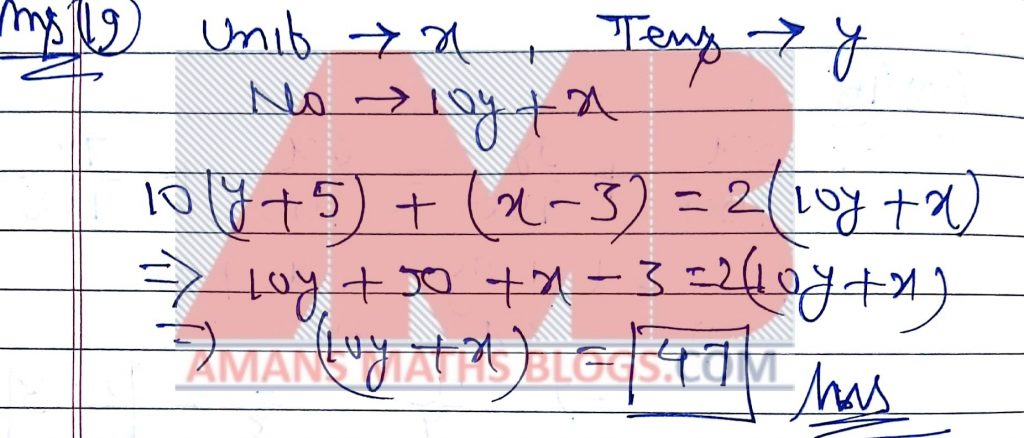NMTC 2018 Paper For Sub Junior Level Ques No 20:

The unit digit of 52018 – 32018 is

Options:

A. 5

B. 6

C. 7

D. 4

Solution: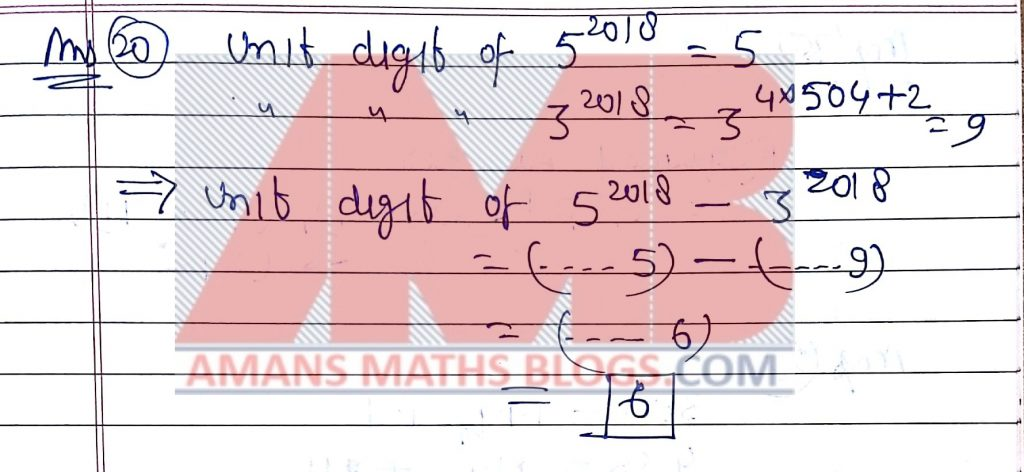Part B: Instruction:

Write the correct answer in the space provided in the responsive sheet. For each correct response, you get 1 mark. For each incorrect response, you lose 1/4 mark.

NMTC 2018 Paper For Sub Junior Level Ques No 21:

The smallest natural number that has to be added to 803642 to get a number which is divisible by 9 is _______.

Solution: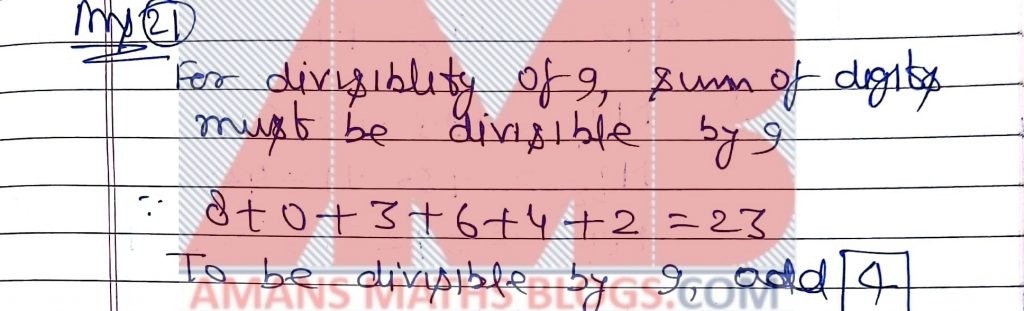NMTC 2018 Paper For Sub Junior Level Ques No 22:

The greatest two digit number that will divides 398, 436 and 542 leaving respectively 7, 11 and 15 as remainder is ____.

Solution: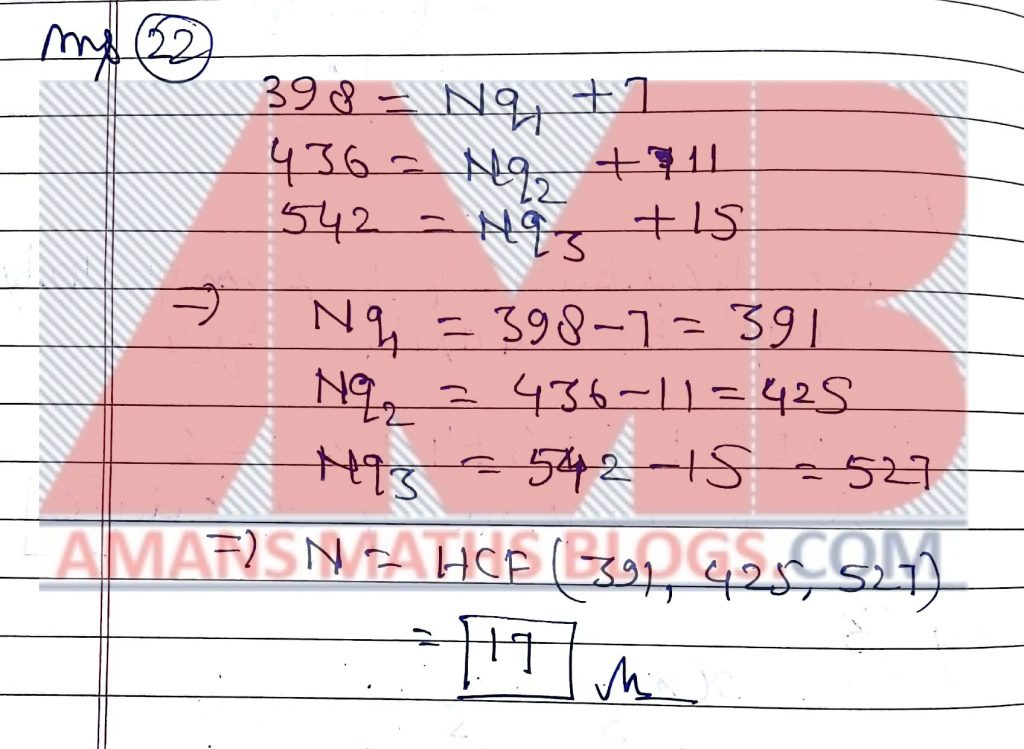NMTC 2018 Paper For Sub Junior Level Ques No 23:

2/3 is _____ of 1/3

Solution: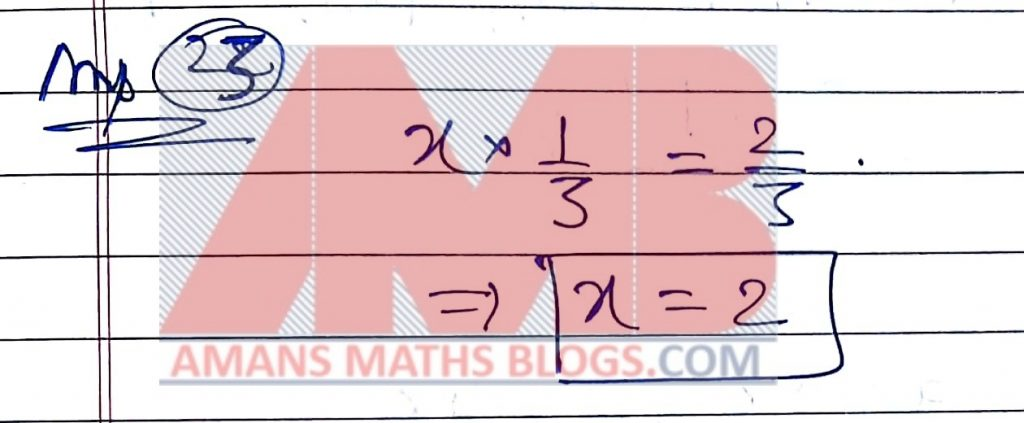NMTC 2018 Paper For Sub Junior Level Ques No 24:

The sum of 5 positive integers is 280. The average of the first 2 numbers is 40. The average of the third and fourth number is 60. The fifth number is ______.

Solution: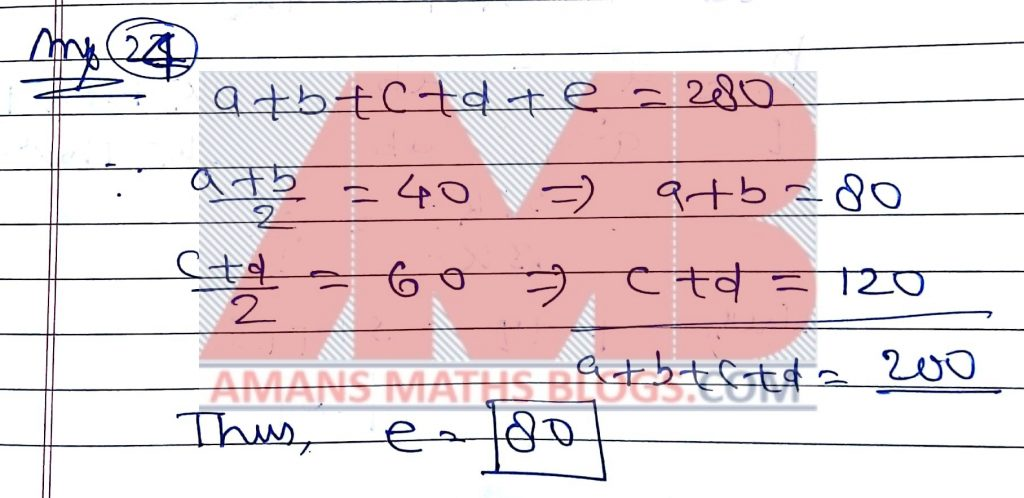NMTC 2018 Paper For Sub Junior Level Ques No 25:

If a : b = 3 : 4 and p/q = (a2 + b2 + ab)/(a2 + b2 – ab), where p, q have no common divisors other than 1, p + q is _____.

Solution: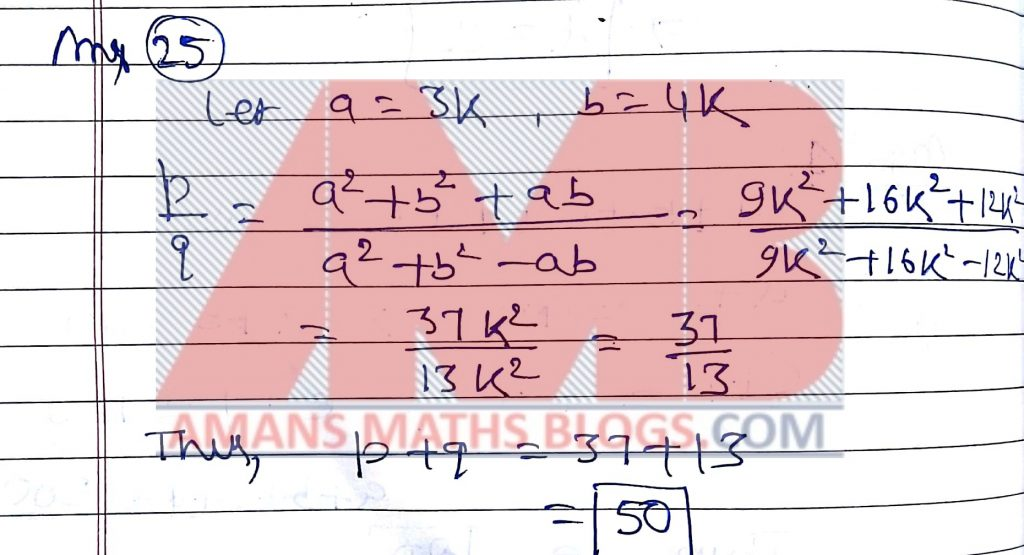NMTC 2018 Paper For Sub Junior Level Ques No 26:

a is a natural number such that a has exactly two divisors and (a + 1) has exactly three divisors. The number of divisors of (a + 2) is

Solution:NMTC 2018 Paper For Sub Junior Level Ques No 27:

The first term of a series is 2/5. If x is a term of this series, the next term is (1 – x)/(1 + x). If tn denotes the nth term and t2018 – t2017 = p/q, where p, q are integers having no common factors other than 1, p + q is ______.

Solution:NMTC 2018 Paper For Sub Junior Level Ques No 28:

In the adjoining figure, the sides of the square is. The area of unshaded region isA sq cms. The value of A is ____________.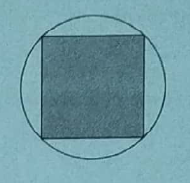Solution: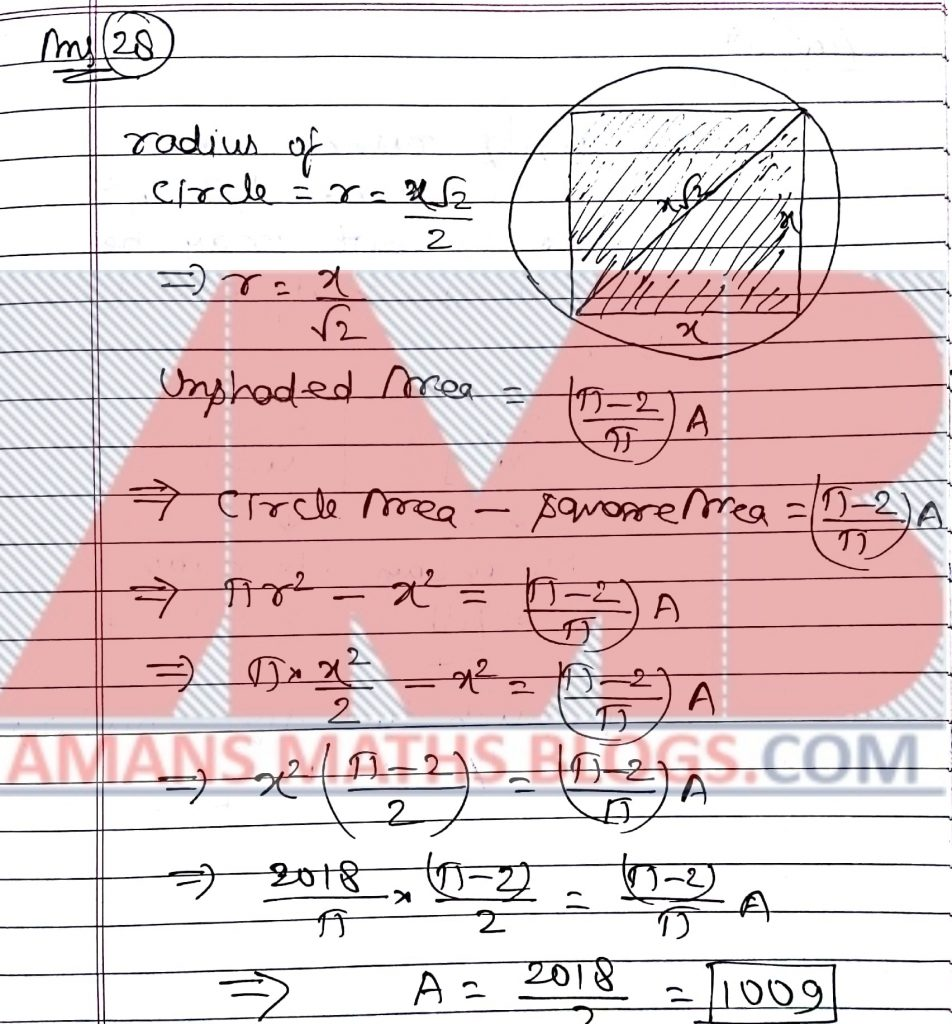NMTC 2018 Paper For Sub Junior Level Ques No 29:

n is natural number. The square root of the sum of the square of n and 19 is equal to the next natural number to n. The value of n is _______.

Solution:NMTC 2018 Paper For Sub Junior Level Ques No 30:

Using only the digits 1, 2, 4, 5 two-digit number are formed. The digits of the two digit number may be same of different digits. The number of such two-digit number is _____.+

Solution:AMBiPi
error: Content is protected !!